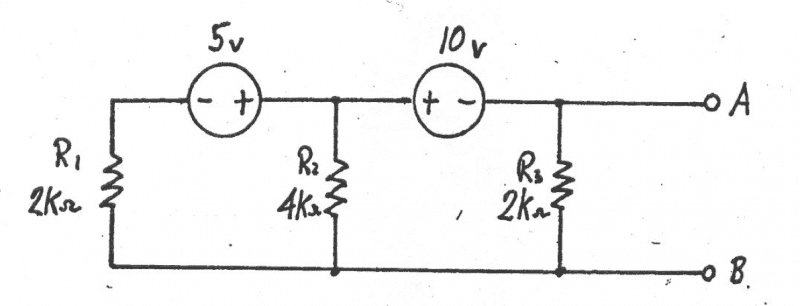# Thevenin's Theorem with Source Transforms

Homework Helper

## Homework Statement

I was able to find the Thevenin equivalent between ##A## and ##B## using loop analysis and then Ohm's law:The Thevenin equivalent was ##V_{th} = -4.0 V## in series with ##R_{th} = 0.8 k##.

Now I had a question about solving the problem using a different approach. I realized now source transforms are a sort of "corollary" to Thevenin and Norton's theorems. So I was wondering if it was possible to use those in solving this problem.

## The Attempt at a Solution

From what I can understand, first I should use ##V = IR \Rightarrow I = \frac{5}{2} mA##. Then I could have a current source in parallel with the ##2k## on the left.

Now ##R_{eq} = (\frac{1}{2k} + \frac{1}{4k})^{-1} = \frac{4k}{3}##.

So I have that ##\frac{5}{2} mA## in parallel with ##\frac{4k}{3}##.

From this point I have not been able to continue using source transforms to simplify to the correct answer. Is it just not possible? Or is it a combination I'm not seeing?

I see that superposition would be useful from this point as well, but I want to know if it's possible with source transforms.

Last edited:

## Answers and Replies

Gold Member
So I have that ##\frac{5}{2} mA## in parallel with ##\frac{4k}{3}##.

From this point I have not been able to continue using source transforms to simplify to the correct answer. Is it just not possible? Or is it a combination I'm not seeing?

I see that superposition would be useful from this point as well, but I want to know if it's possible with source transforms.

Convert ##\frac{5}{2} mA## in parallel with ##\frac{4k}{3}## back into a voltage source of 10/3 volts in series with a resistance of 4000/3 ohms. Now you have 2 voltage sources in series with two resistors. Slide the 4000/3 ohm resistor to the right so it's just above the 2k output resistor. Those two resistors form a voltage divider driven by the difference in the two voltage sources.

I get Vth = -4V

•STEMucator
Homework Helper
Convert ##\frac{5}{2} mA## in parallel with ##\frac{4k}{3}## back into a voltage source of 10/3 volts in series with a resistance of 4000/3 ohms. Now you have 2 voltage sources in series with two resistors. Slide the 4000/3 ohm resistor to the right so it's just above the 2k output resistor. Those two resistors form a voltage divider driven by the difference in the two voltage sources.

I get Vth = -4V

I tried this earlier, but I messed the numbers up and it didn't spit the right answer out.

Reading your post and going back to it, I face-palmed and realized my mistake. I now get the same answer in 10 different ways so I should be good.

Thank you.